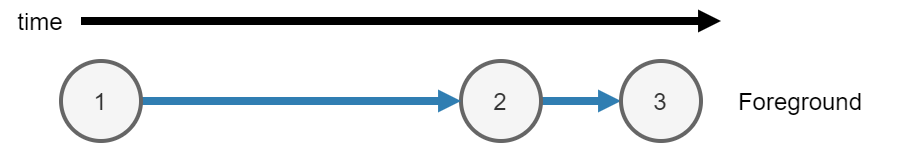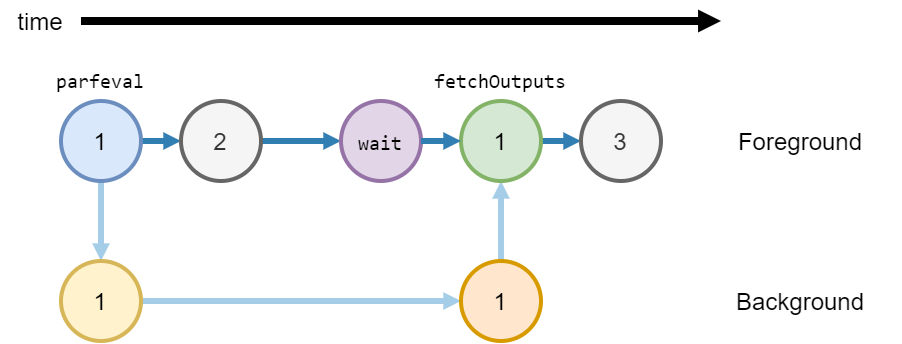## Asynchronous Functions

MATLAB® either runs code synchronously or asynchronously. You can use the following functions to run code asynchronously:

Calculate the maximum of two random matrices. MATLAB runs each line consecutively.

```tic A = rand(10000); B = ones(10000); C = max(A,B); toc```
`Elapsed time is 0.992156 seconds.`

When you run the code, MATLAB runs three calculations.

1. Calculate `A = rand(10000)` in the foreground.

2. Calculate `B = ones(10000)` in the foreground.

3. After creating `A` and `B`, calculate ```C = max(A,B)``` in the foreground.### Asynchronous Code

When you run a function asynchronously, MATLAB can run other code in the foreground at the same time.

Use `parfeval` or `parfevalOnAll` to run functions asynchronously. Use `afterEach` and `afterAll` to run functions asynchronously after a previous function completes.

Synchronous FunctionsAsynchronous Functions
MATLAB waits for the function to complete.MATLAB does not wait for the function to complete.
MATLAB runs the code immediately.MATLAB runs the code when a worker is available. For more information, see Background Workers.
Outputs from the function are available in the current workspace.To copy outputs to the current workspace, use `fetchOutputs`. When you use `fetchOutputs`, MATLAB waits for the function to complete.
You can use any function or object in a synchronous function.

You can use most functions and objects in an asynchronous function.

• Any function or object that has thread support will run in the background or on a `ThreadPool`. For more information, see Run MATLAB Functions in Thread-Based Environment.

• All functions and objects are supported on process-backed parallel pools (`ProcessPool` and `ClusterPool`). For more information, see Run Code on Parallel Pools (Parallel Computing Toolbox).

The current workspace is also available in a synchronous function.Most of the variables in the current workspace are also available in an asynchronous function.

Calculate the maximum of two random matrices: one created in the background, and one created in the foreground. Matrix `A` is created in the background, and matrix `B` is calculated in the foreground at the same time.

Note

Generally, you do not need to use `wait`. `fetchOutputs` will implicitly wait for MATLAB to finish running the function in the background before collecting results. The function `wait` is used here to explicitly show waiting for results before collecting them.

```tic fA = parfeval(backgroundPool,@rand,1,10000); B = ones(10000); wait(fA) C = max(fetchOutputs(fA),B); toc```
`Elapsed time is 0.534475 seconds.`

When you run the code, MATLAB runs three calculations.

1. Calculate `A = rand(10000)` in the background.

1. Use `parfeval` to schedule the function `rand` to run in the background, with `1` output and a single input `10000`. Return a future `fA` in the foreground.

2. Run the function `rand` in the background.

2. Calculate `B = ones(10000)` in the foreground.

3. After creating `A` and `B`, calculate ```C = max(A,B)``` in the foreground.

1. Use `wait` to explicitly wait for the future `fA` to finish running in the background.

2. Use `fetchOutputs` to get `rand(10000)` from the future `fA`.

3. Calculate the final result `C` from matrices `fetchOutputs(fA)` and `B`.### Background Workers

When you use `parfeval` or `parfevalOnAll` to run a function asynchronously, MATLAB runs the function on a pool.

When you use `backgroundPool` to run code in the background, MATLAB uses the background pool to run that code.

The background pool has a fixed number of workers. MATLAB uses these workers to run functions. Each worker can only run one function at a time. Therefore when you run multiple functions in the background, you must wait for a worker to be available to run each function.

Use the `NumWorkers` property of a `BackgroundPool` to find out how many workers you have.

• If you do not have Parallel Computing Toolbox, the background pool has `1` worker.

• If you have Parallel Computing Toolbox, the background pool has multiple workers. The number of workers in the background pool is `min(8,N)` where `N` is the number of physical cores on your machine. For example if your machine has 4 cores, the background pool has `4` workers. If your machine has 16 cores, the background pool has `8` workers.